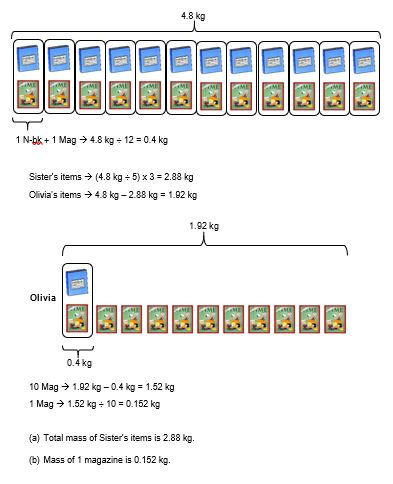# Question

Olivia bought 12 identical magazines and her sister bought 12 identical notebooks. The total mass of all the items Olivia and her sister bought was 4.8 kg. Olivia then exchanged a magazine with her sister for a notebook. After the exchange, the total mass of the items Olivia  had was 2/3 of the total mass of the items her sister had.

a) Find the total mass of all the items Olivia’s sister had after the exchange.

b) Find the mass of a magazine.

3 Answers

# Answer0 Replies 2 Likes ✔Accepted Answer

12 identical magazines + 12 identical notebooks ——- 4.8
1 identical magazine + 1 identical notebook ——- 0.4
1 identical magazine ——- 0.4 – 1 identical notebook
(11 identical magazines + 1 identical notebook)/(1 identical magazine + 11 identical notebook) = 2/3
33 identical magazines + 3 identical notebooks = 2 identical magazines + 22 identical notebooks
31 identical magazines = 31 x (0.4 – 1 identical notebook) = 12.4 – 31 identical notebooks ——- 19 identical notebooks
1 identical notebook ——- 12.4/(19 + 31) = 0.248
1 identical magazine ——- 0.4 – 0.248 = 0.152 (b)
11 x 0.152 + 0.248 = 1.92 (a)

Ans : (a) 1.92 kg; (b) 0.152 kg.

0 Replies 0 Likes